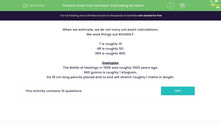# Estimating

In this worksheet, students answer a selection of mixed questions in which they must use estimation.Key stage:  KS 2

Curriculum topic:   Number: Number and Place Value

Curriculum subtopic:   Represent Numbers to 1000

Popular topics:   Estimation worksheets

Difficulty level:#### Worksheet Overview

In this activity, we will be learning about using estimating in maths.

When we estimate, we do not carry out exact calculations.

We work things out using approximate values.

To do this, we might round a number up or down to the closest multiple of 10 or 100.

7 and 11 are both approximately 10

48 and 51 are both approximately 50.

389 and 403 are both approximately 400.

So, if there are 396 jelly beans in a jar and Simon has 2 jars. How many beans does he have altogether?

396 is very close to 400.

400 is much easier to double than 396.

400 × 2 = 800.

So, we could say he has approximately 800 jelly beans

Let's try an example question together.

Example

Benny's socks are 19cm, 17cm, 21cm, and 22cm long.

He lays them out on a line.

Approximately how long is the line?

Benny's socks are all approximately 20cm long.

He has 4 pairs of socks.

20 × 4 = 80

The line of socks will be approximately 80cm long.

Now you've seen estimating in action, it's time to try some questions by yourself.

### What is EdPlace?

We're your National Curriculum aligned online education content provider helping each child succeed in English, maths and science from year 1 to GCSE. With an EdPlace account you’ll be able to track and measure progress, helping each child achieve their best. We build confidence and attainment by personalising each child’s learning at a level that suits them.

Get started